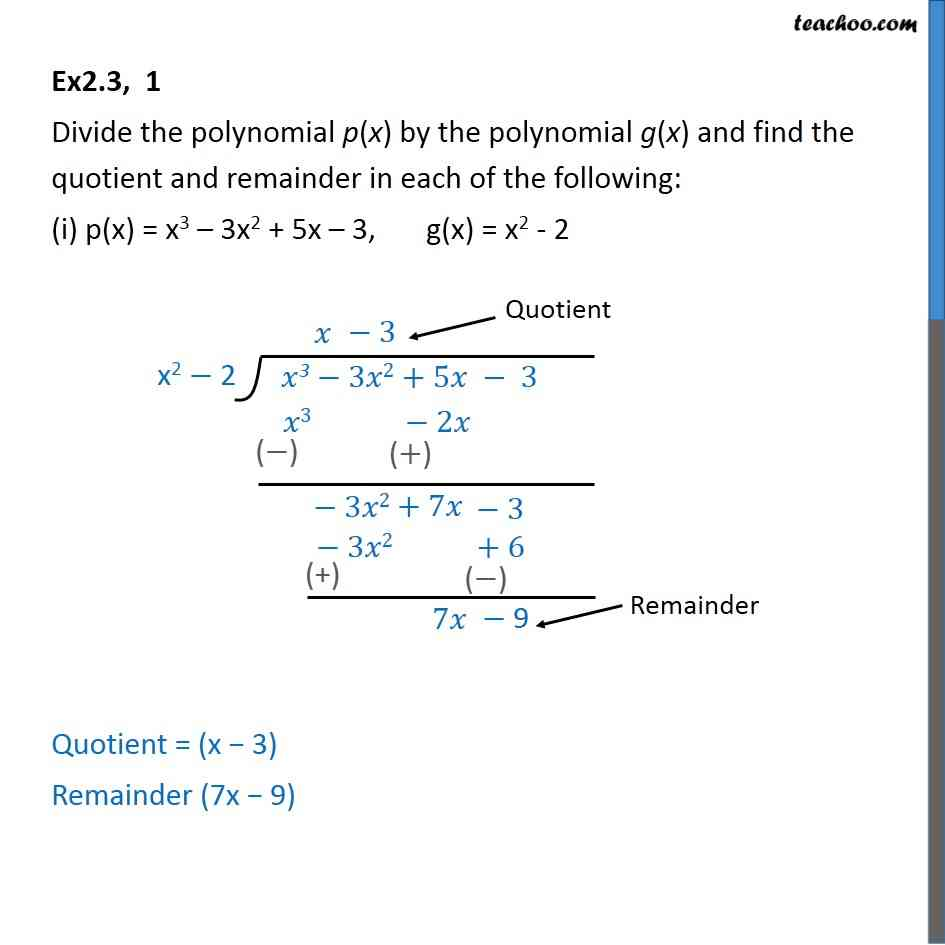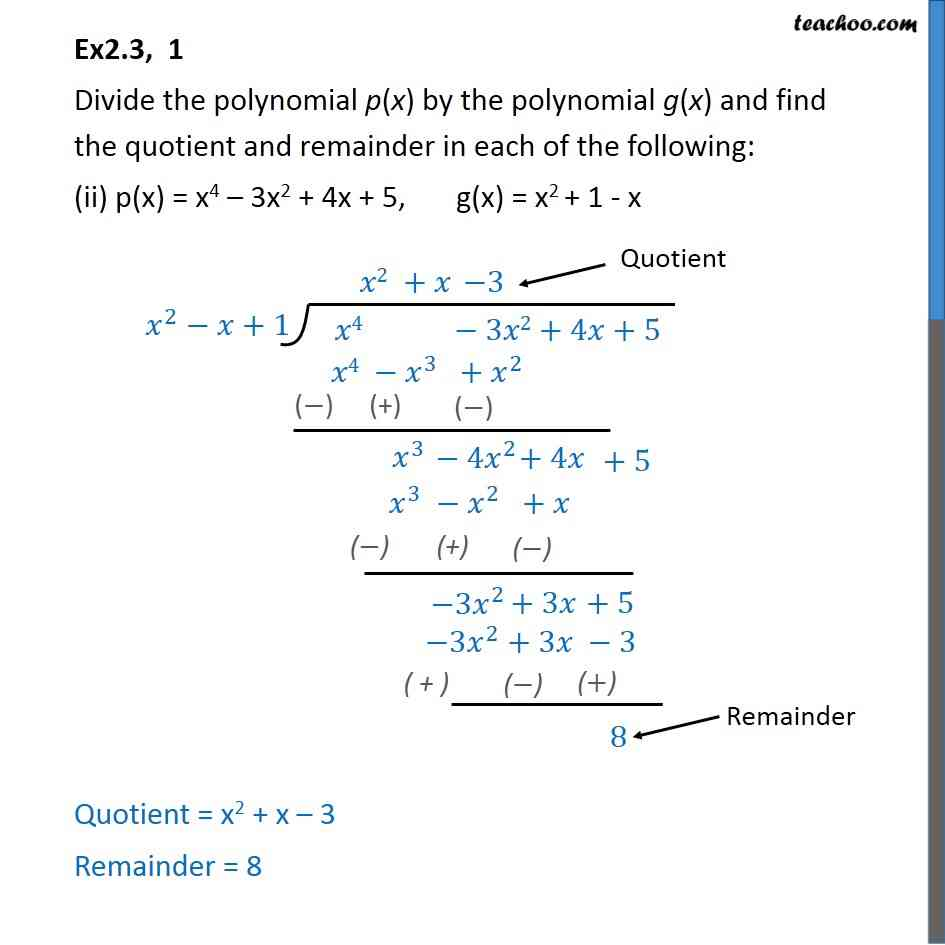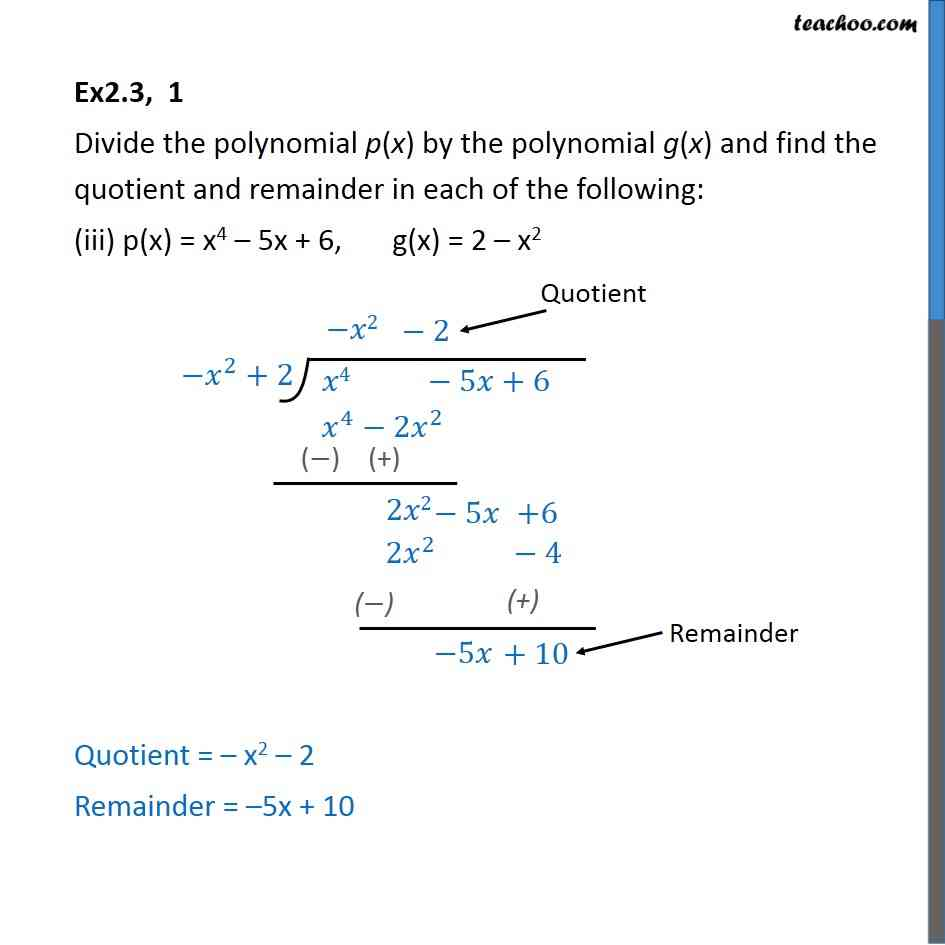Learn Polynomials - Start to Finish - FREE. Check - Polynomials Class 101. Chapter 2 Class 10 Polynomials
2. Serial order wise
3. Ex 2.3

Transcript

Ex2.3, 1 Divide the polynomial p(x) by the polynomial g(x) and find the quotient and remainder in each of the following: (i) p(x) = x3 – 3x2 + 5x – 3, g(x) = x2 - 2 Quotient = (x − 3) Remainder (7x − 9) Ex2.3, 1 Divide the polynomial p(x) by the polynomial g(x) and find the quotient and remainder in each of the following: (ii) p(x) = x4 – 3x2 + 4x + 5, g(x) = x2 + 1 - x Quotient = x2 + x – 3 Remainder = 8 Ex2.3, 1 Divide the polynomial p(x) by the polynomial g(x) and find the quotient and remainder in each of the following: (iii) p(x) = x4 – 5x + 6, g(x) = 2 – x2 Quotient = – x2 – 2 Remainder = –5x + 10

Ex 2.3

Chapter 2 Class 10 Polynomials
Serial order wise